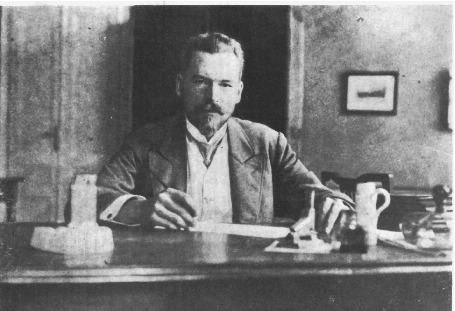#32nd M. Smoluchowski Symposium on Statistical Physics

18-21 September 2019
Kraków
Europe/Warsaw timezone

## Kinetic equation for the pair distribution function \\ in the Boltzmanns gas

Not scheduled
15m
Kraków

Poster

### Speaker

Dr. Ryszard Wojnar (Instytut Podstawowych Problemów Techniki PAN)

### Description

We report on a new kinetic equation for an auxiliary two-particle distribution function
$f({\bf k_1}, {\bf v_1}, {\bf k_2}, {\bf v_2}, t)$.
\begin{equation}
\begin{array}{l}
\displaystyle
\left(\frac{\partial}{\partial t} \, + \, {\rm i} \, {\bf k_1} {v_1} \, + \, {\rm i} \ {\bf k_2} {v_2} \right)
f({\bf k_1}, {\bf v_1}, {\bf k_2}, {\bf v_2}, t) \, =
\
\displaystyle
\hspace{5cm}
\int_0^t {\rm d} \tau \, {\cal G}(({\bf k_1}, {\bf k_2}, \tau) \, f({\bf k_1}, {\bf v_1}, {\bf k_2}, {\bf v_2}, t - \tau)
\end{array}
\end{equation}
with
\begin{equation}
\begin{array}{l}
\displaystyle
{\cal G}(({\bf k_1}, {\bf k_2}, \tau) \, \equiv \,
\
\displaystyle
\hspace{2cm}
\int
{\rm d} {\bf v}^{N-2} \, {\rm d} {\bf r}^N \, {\rm e}^{- \,{\rm i} {\bf k_1}{\bf r_1}} \, {\rm e}^{- \,{\rm i} {\bf k_2}{\bf r_2}} \,
{\cal P} K_N \,
\
\displaystyle
\hspace{3.5cm}
{\rm e}^{- \, \tau \, (1 - {\cal P}) K_N } \,
{\rm e}^{{\rm i} {\bf k_1}{\bf r_1}} \, {\rm e}^{{\rm i} {\bf k_2}{\bf r_2}} \,
\frac{f^0_N ({\bf v}^N, {\bf r}^N)}{\varphi_{\rm M}(v_1) \, \varphi_{\rm M}(v_2)}
\end{array}
\end{equation}
which is the scattering operator for our problem.
We have applied to this problem the technique of projection operators.
We find that ${\cal G}(t)$ is finite at $t= 0$ and can be readily expanded in Taylor series about $t= 0$. This is a distinct feature which is not present usually in other kinetic equations. Therefore the kinetic equation (1) with tne kernel (2) is not only valid for long times but for arbitrarily short times, including $t= 0$, as well.

Next we set the distance between particles 1 and 2, $| {\bf r} _1 - {\bf r} _2 | = a$ = constant. This creates a dumbbell model of molecule, the diffusion of which is being studied.

### Primary author

Dr. Ryszard Wojnar (Instytut Podstawowych Problemów Techniki PAN)

### Presentation Materials

There are no materials yet.
###### Your browser is out of date!

Update your browser to view this website correctly. Update my browser now

×